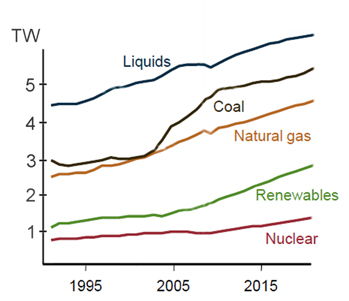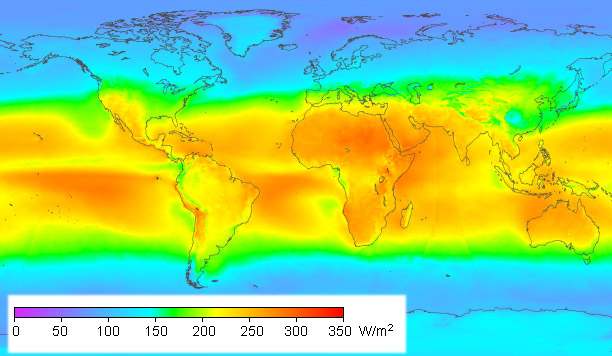# Solar Energy

I have been looking into the subject of renewable energy, and trying to get its potential into perspective. Can it really replace fossil fuels; or else are we facing the crunch of peak oil predicted since the 1970's ? The graph below is derived from US Department of Energy data, and shows the record of energy consumption by source. Their graph of energy from liquids ( oil ) shows a steady rise - no sign of peak oil here.global energy sources - from US dept of energy figures

This graph measures energy sources in Terawatts (TW), which are equal to a million Megawatts (MW). The total energy we get from oil is equivalent to the continuous, round the clock generation of about 5 TW. Now let's get some basic figures from wikipedia. Crude oil has a chemical energy  of 1.7 MWh per barrel. We can also use the standard unit if energy which is Joules, but I like to think in barrels of oil because I can visualise that more easily. I see a big round barrel which is 42 gallons, or a couple of tanks filling an average car. Oil production is measured in barrels per day (bpd). So one barrel of oil per day (bpd) is 1.7MWh / 24h = 71KW. To generate 5TW we need to burn 5TW / 71KW = 70 million bpd.

There are other figures on wikipedia that give global oil production as more than 70m bpd but then oil is used for other things as well such as plastics and lubricants. So the figures are consistent. What potential does renewable energy have to replace this ? If we add together oil, coal, gas and nuclear energy the total is around 15TW. That's the target for proponents of renewable energy.

## bio-fuel

This is a controversial energy source, which according to its critics involves converting perfectly good food into a relatively tiny amount of gasoline. How tiny exactly ? Soybean yields on average  are about 1000kg per acre per year. We normally measure the energy of food using calories (kcal). This is the energy required to heat one kilo of water by one degree C, and is equivalent to 4.2KJ. The calorific content of soya is given  as 4400kcal per kg. So the acre crop of soya yielding 1000kg has an energy value one thousand times that, or 4.4M kcal. Which is 4.4M x 4.2KJ = 18000MJ approx.

Now a MWh, in joules, is 1,000,000 Watts x 3600 seconds = 3600MJ. Hence the tonne of soya is 18000MJ / 3600MJ = 5 MWh. Then the oil equivalent of the tonne of soya is 5 MWh / 1.7MWh = 3 barrels of oil approx.

The food energy of 4400kcal ( a kilo of soya ) is enough to feed a man for a day, so we could also think of the tonne of soya as feeding a man for 1000 days, or perhaps a family for a year. So that is the comparison of food to fuel : feed a family for a year or get 3 barrels of gasoline.

Whether that is a reasonable yield depends on your point of view. However, let's now consider the total fuel demand of 70m bpd. That is 70m bpd x 365d = 25 billion barrels. To produce that from soya we need 25 billion barrels / 3 barrels per acre of soya = 8 billion acres under cultivation. There are 640 acres in a square mile, so that area is 8 billion / 640 = 12 million square miles. That is almost a quarter the land area of the earth and more than the total amount of arable land. So it's not practical to replace fossil fuels with biofuel given the sort of crops like soy that we are currently using.

Could there there some other crop, perhaps genetically engineered, that might yield much more ? Biofuel ultimately depends on the energy of sunlight trapped through photosynthesis. Plants like soy are being used like organic solar panels. How efficient is this compared to man-made panels ? That's the next question I want to answer.

## solar power

The graphic below shows how much solar power reaches the earth's surface, measured in Watts per square metre, averaged over a year - day and night.solar insolation - from work by Matthias Loster

An acre of land is 4000 sqm, and the central USA is getting about 200W / sqm, so the acre gets an average power of 800KW from the sun. That's quite a lot. That's 19MWh in a day, equivalent to about 11bpd of oil. Compare that with the biofuel acre, which gives us a meagre 3 barrels per year. Put another way, the soybean crop yields less than one thousandth of the energy potential of the sun. For me the issue with biofuel is not that it pushes up the price of food, but that it's such a poor technology for generating power.

We can do much better with solar power. The generators operate in one of two ways. Solar concentrators work by focusing sunlight onto a boiler. The principle is simple enough, but they are useless on a cloudy day. The alternative is photovoltaic cells - commonly known as solar panels. These work with light from any direction - including light scattered by clouds. Both technologies can convert a reasonable percentage of the energy of sunlight into electricity - the best installations reaching about 20-30% efficiency . So could this generate the 15TW we currently get from fossil fuels ? The answer, surprisingly, is yes. In a sunny location like the southwestern USA, assuming sunlight of 250 W/m2 and 20%conversion efficiency, you need to cover 300,000 km2 with panels to generate an average 15TW. That is the land area of the state of Arizona - to generate enough power for the whole world.

Engineering on that scale might seem like science fiction, but then consider the Herculean efforts already being made by the oil industry to find new deposits. From this point on, it only gets harder for them. We are getting to the point where a Watt of solar power costs about the same as energy from oil and other non-renewable sources. If we spent as much money on renewables as we spend on fossil fuels then we could conceivably build a sustainable energy infrastructure. There is a further challenge, beyond just installing solar panels : to store and transport the energy, and to convert it to the required form. But it's basically the same problem faced by any source of energy. Natural gas, for instance, might be sourced in the middle east, liquefied, shipped to a terminal in Europe, piped to a power station, and burnt to generate electricity, which is then transmitted at high voltage and converted to low voltage for a home user. The cycle for solar power might involve using electricity to generate hydrogen from water, then shipping the hydrogen around as a fuel. Yes it's a lot of effort, but once the infrastructure is in place your energy problems are solved permanently. Contrast that with the oil industry, which is constantly on the move to get to new deposits, and pollutes the environment wherever it goes. The potential is there for solar power - and the revolution is just beginning.

 wikipedia 'Barrel of oil equivalent' gives 1.7MWh per barrel

 wikipedia 'Bushel' gives one bushel of soya as 27kg; USDA shows typical soybean yields around 40 bushels / acre; combining we get 40 x 27 = 1080 kg / acre

 wikipedia 'Soybean' gives 100g of soya as 440 kcal approx, which is 4400 kcal / kg

 wikipedia 'Solar cell efficiency'